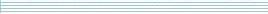| home      | people     | research     | publications    | seminars    | events     | contactCommunication Networks   | Systems Biology   | Hybrid Systems    | Machine Learning   | Dynamics & Interaction### Schools Mathematics Grand Challenge

PROBLEM 16:

There is a well known story told about Carl Friedrich Gauss, a famous German mathematician. On his first day at primary school, his teacher wanted a break, and so decided to ask the students to add up all of the whole numbers from 1 to 100 inclusive. However, the teacher's break was shorter than he had hoped as Gauss replied with the correct answer almost immediately. What he noticed was that the sum (1 + 100) + (2 + 99) + (3 + 98) + .... + (100 + 1) was equal to twice the desired sum. But this sum is simply 100 multiplied by 101, which is 10100. So the answer is half of this or 5050.

Inspired by this story, in this problem we are asking for the sum of all of the digits of all of the whole numbers from 1 to 1,000,000 (inclusive).

NOTE: For example, the sum of the digits of all of the whole numbers from 15 to 21 inclusive would be

(1+5)+(1+6)+(1+7)+(1+8)+(1+9)+(2+0)+(2+1) = 45.

PROBLEM 17:

The rectangular field in the figure below above has been divided into several smaller fields. Each of the different coloured smaller fields is a square. If the length of the side AB is 32 metres, what is the length, in metres, of the side AD?NOTE: The diagram is simply to illustrate the problem. It is not to scale.

PROBLEM 18:

Mary had a very strange day last Monday. First of all, her mother asked her to choose a prime number between 100 and 200, and then multiply it by itself. Mary did this and told her mother the answer. Her mother then divided Mary's answer by 8, found the remainder and then gave Mary that exact number of 10 euro notes. For example, if the remainder was 2, then Mary got 20 Euro.

As if this wasn't odd enough, Mary was then sent by her mother to a very strange shop, which sells ropes. The shop's owners never liked fractions at school, so they only sell ropes whose length is a whole number of metres (m), but they stock all whole lengths from 1m to 2000m. The shop has an even more curious pricing scheme, the rules of which are:

1. The price of a rope is determined by its length;
2. These is a deal on rope whose length (in metres) has 3 as its units digit - it is free;
3. The total price of any two ropes is the same as the price of another rope whose length is the product of their lengths. So for instance, two ropes of length 2m and 3m would cost exactly the same as a rope of length 6m.

Mary bought a rope whose length (in metres) was equal to the prime number she chose. If the only money Mary had was that given her by her mother, how much change in euro did she have when she left the shop?

NOTE: In this shop, just because a rope is longer, it does not mean that it is always more expensive. However, they never charge negative prices.